# 数学基础

## 基本初等函数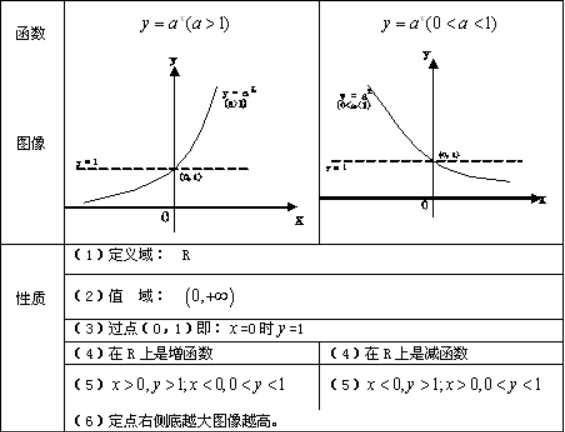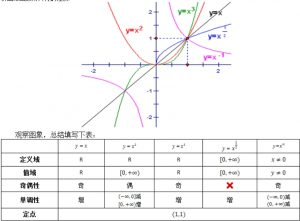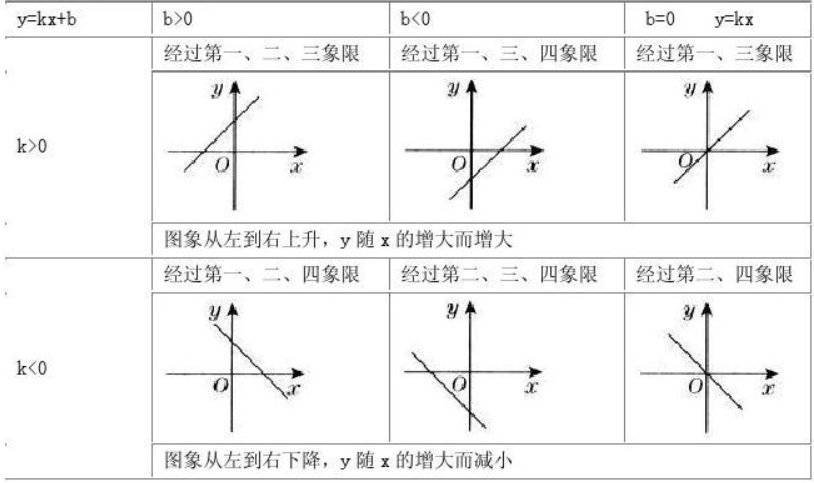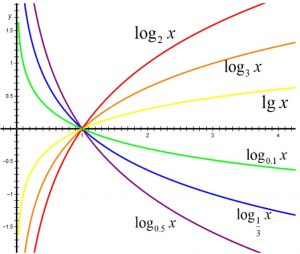## 简单代数计算

### 数数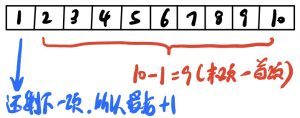### 指数与对数互换

b是以a为底的n次幂。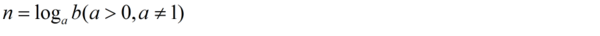OK，数学所需的基础也就这些。普遍都是我们已经很熟悉的东西。

# 算法执行次数T(n)

## 典例一：单层循环

#include <stdio.h>

int main(void) {
for (int i = 0; i<n; i++) {     //执行了n+1次
printf("Hello World!");     //执行了n次
}
return 0;
}

1. 这个程序肯定是无法运行的（变量n未定义），所以我们不运行它。我们先分析循环里面的printf()语句：
2. 根据上面的数学知识，“i从0（含）到n（不含）”等价于“i从0（含）到n-1（含）”——请停下来好好想下，没想通的话不要继续看下面的内容，请留言告诉我。
3. 那么，i从0（含）到n-1（含）共执行了(n-1)-0+1=n次。所以最内层执行了n次。
4. 因为“for (int i = 0; i<n; i++)”执行到最后，当i=n时，虽然不满足i<n的条件。但仍然算执行了一次。——认真想明白后再往后看，如果想不通，请试数（将n取5或任何数，数一数执行了多少次，很显然是6次）

## 典例二：双层嵌套“内外都不变”循环

#include <stdio.h>

int main(void) {
for (int i = 0; i<n; i++) {
for (int j = 0; j<n; j++) {
if (... ...)    //共执行n*n次
}
}
return 0;
}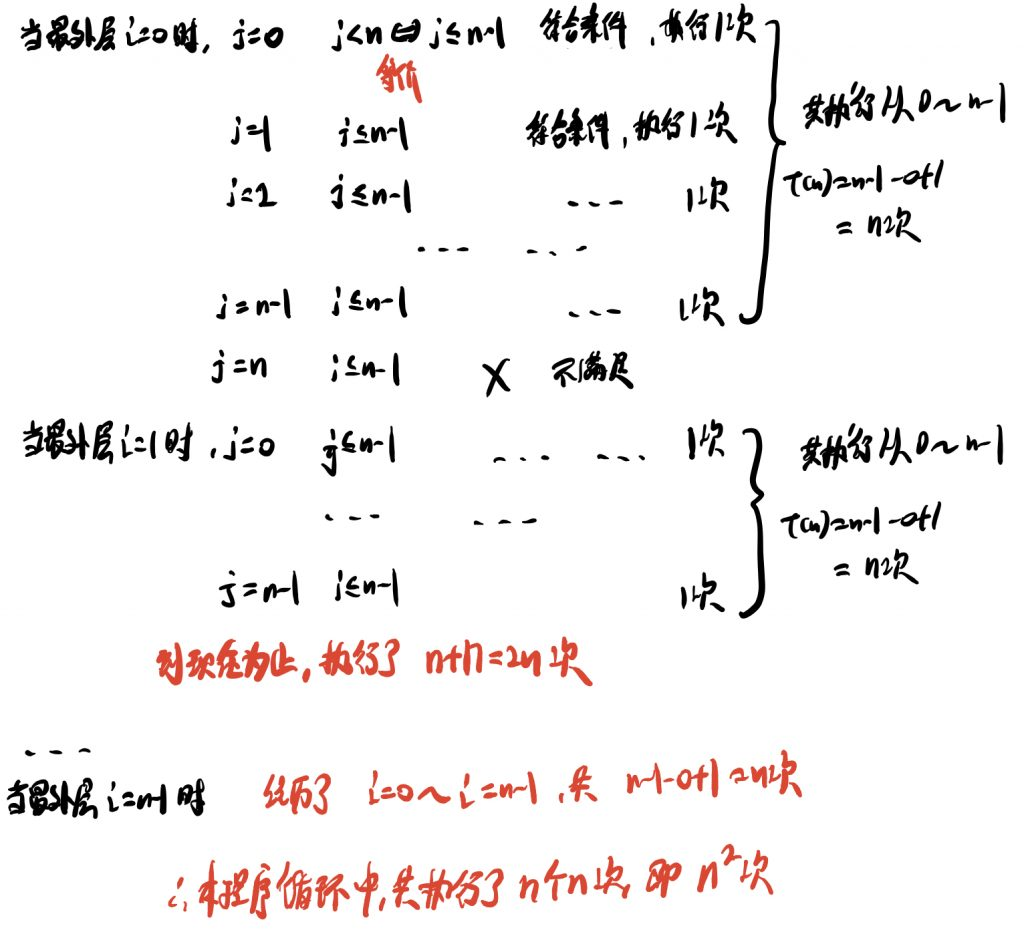## 典例三：双层嵌套“内变外不变”循环

#include <stdio.h>

int main(void) {
for (int i = 0; i<n; i++) {
for (int j = i; j<n; j++) {     //内层j会随着i的改变而自增
... ....
}
}
return 0;
}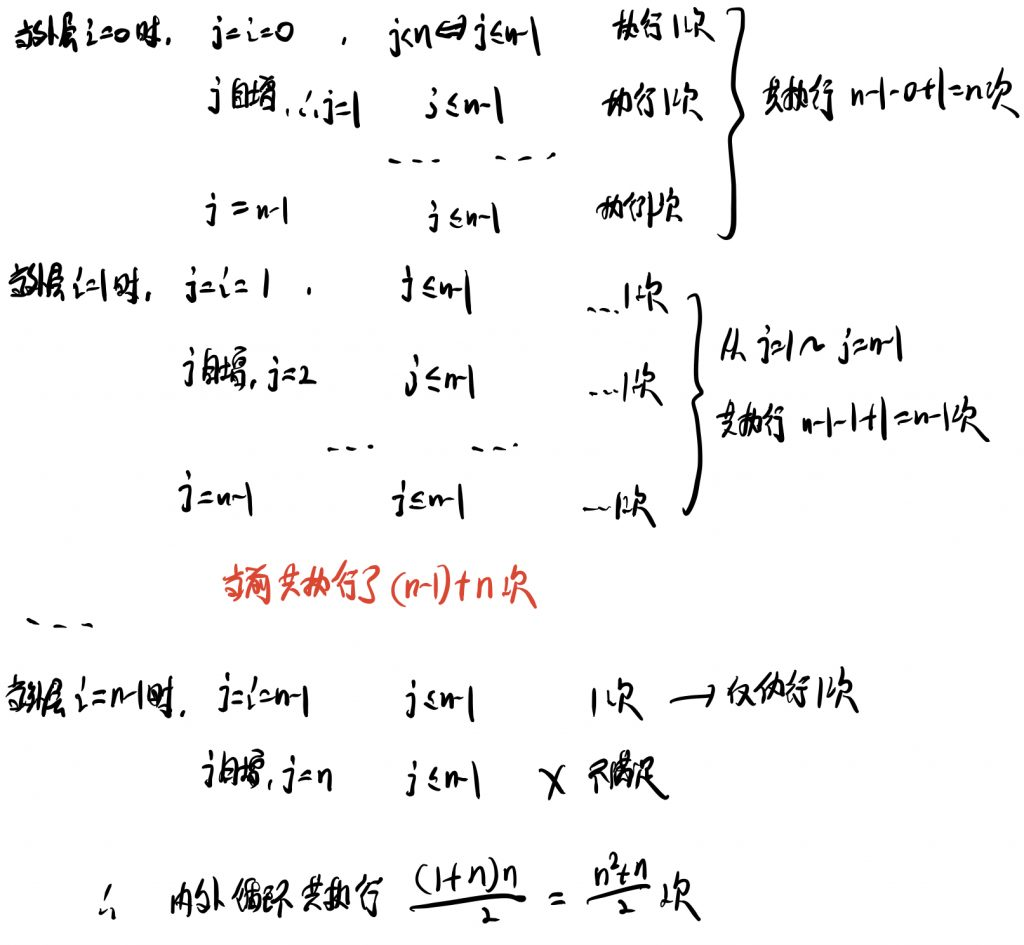## 典例四：循环变量非连续增加或减少式

#include <stdio.h>

int main(void) {
int i=0;
while (i<n) {
i=i+2;
}
return 0;
}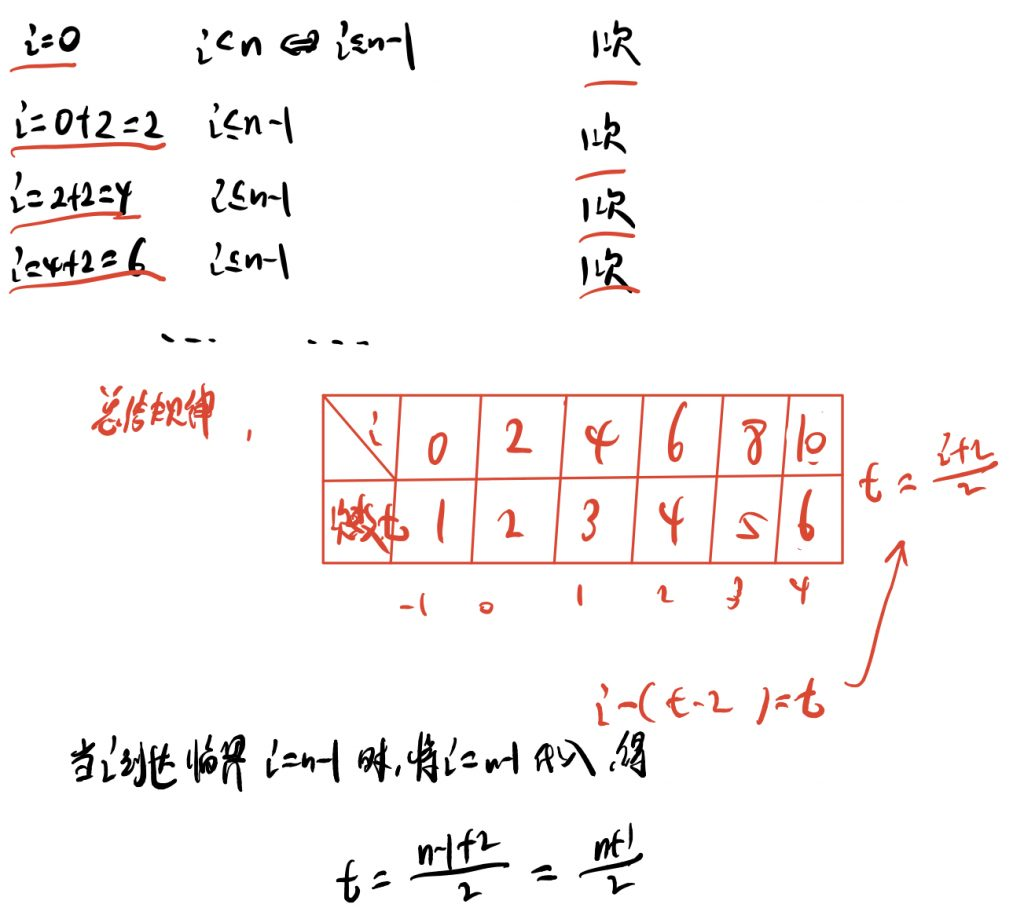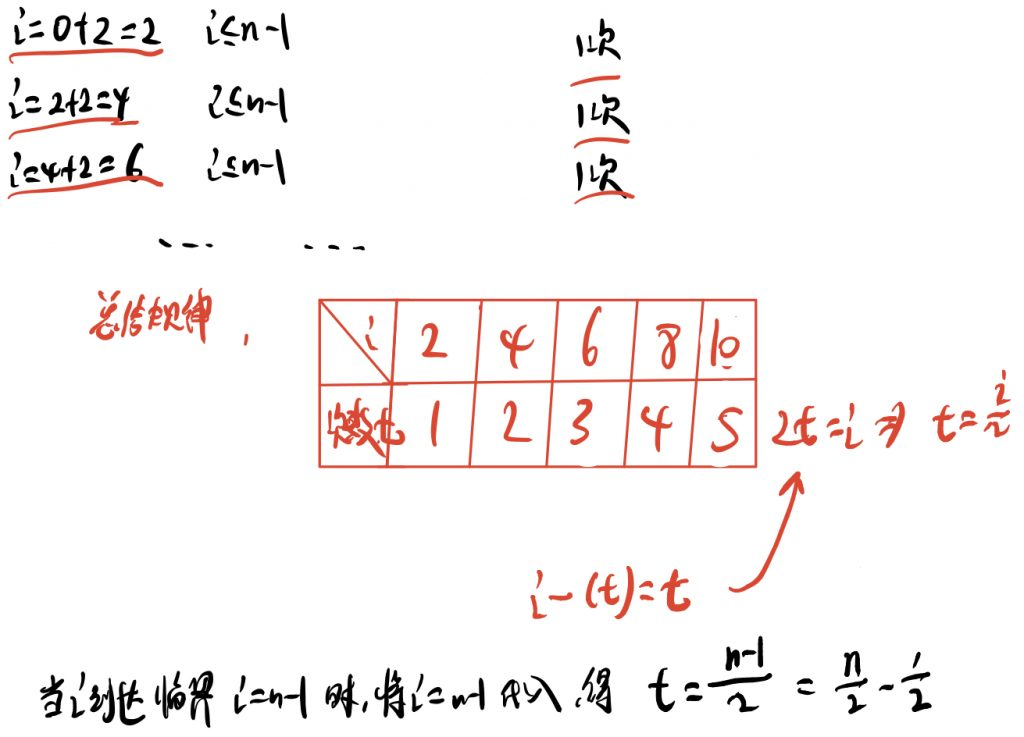## 典例五：循环变量指数增加式

#include <stdio.h>

int main(void) {
int i=0;
while (i<n) {
i=i*2;
}
return 0;
}

#include <stdio.h>

int main(void) {
int i=1;
while (i<n) {
i=i*2;
}
return 0;
}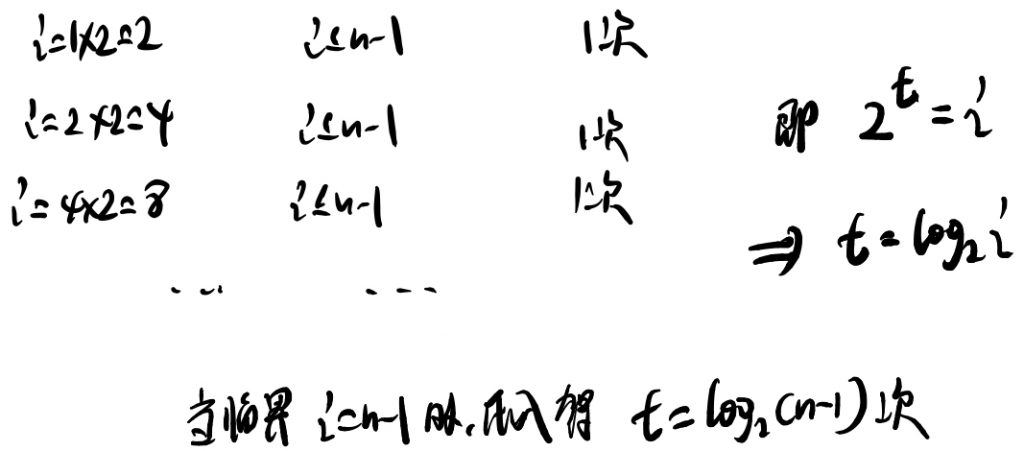## 典例六：顺序执行的语句或者算法

#include <stdio.h>
int main(void) {
for(int i = 0; i < n; i++) {
for(int j = 0; j < n; j++) {
printf("Hello, World!\n");      //执行次数为n^2次
}
}

for(int j = 0; j < n; j++) {
printf("Hello, World!\n");          //执行次数为n次
}
return 0;
}


## 典例七：含有条件判断语句式

#include <stdio.h>
int main(void) {
if (...) {
for(int i = 0; i < n; i++) {
for(int j = 0; j < n; j++) {
printf("Hello, World!\n");      //执行次数为n^2次
}
}
} else {
for(int j = 0; j < n; j++) {
printf("Hello, World!\n");          //执行次数为n次
}
}
return 0;
}

# 函数的渐近增长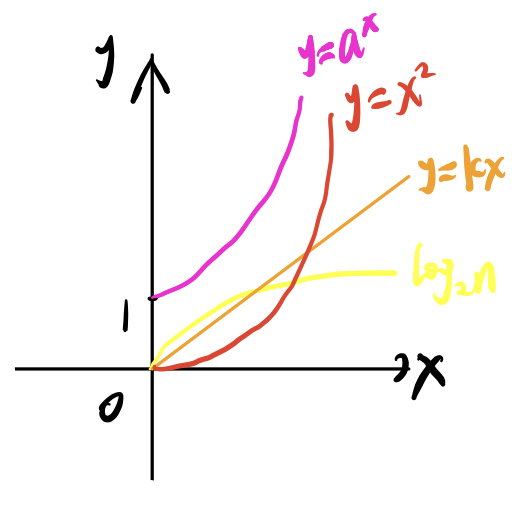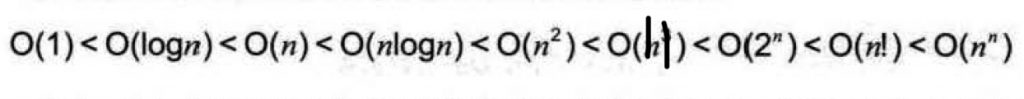# 算法时间复杂度

## 加减法常数

(n+1)/2、(n-1)/2、n/2对比：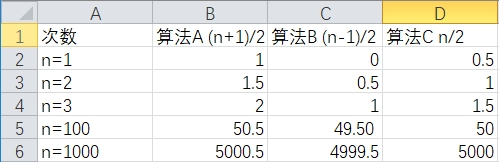## 项后面的常数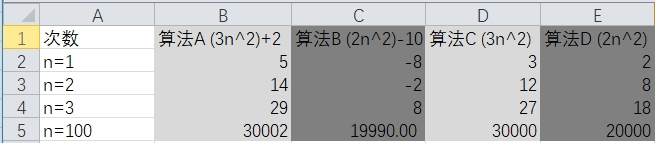## 与n相乘的常数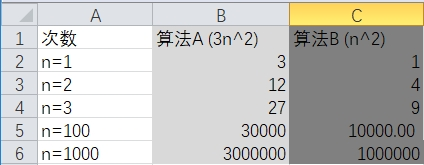1. 我们基本可以分析出：随着问题规模n的增长，某一算法可能越来越优于某种算法，也可能越来越差于某种算法。我们分析时间复杂度时只关心影响变化趋势最大的高阶数。
2. 所以我们又把时间复杂度称为渐进时间复杂度。
3. T(n)是执行次数函数、f(n)是问题规模的某个函数、O(n)是渐进时间复杂度。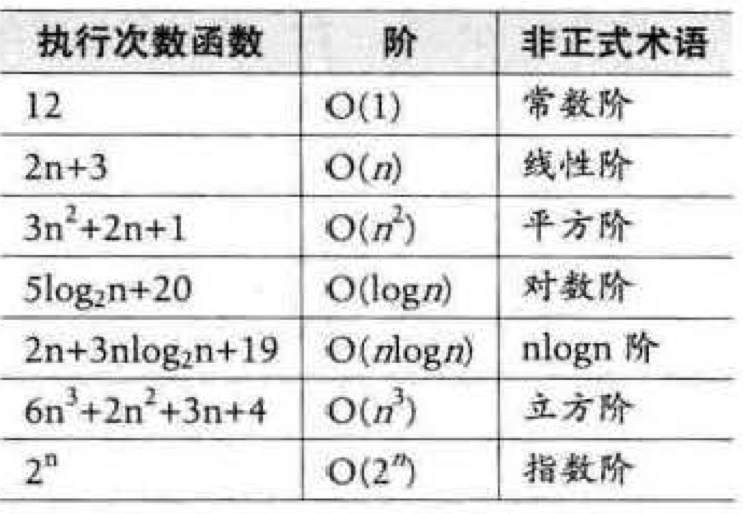# 最坏情况与平均情况